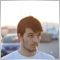# How to get Breakeven point1434

The question is how to get breakeven point for a grid of orders.

I think it could be storing the Ask/Bid Price when OrdersProfit=0 and then apply TP distance to that price.

But if I want to draw a line in that BreakevenPoint, how do I calculate its price?20233

1. David Diez BreakevenPoint, how do I calculate its price?

Volume weighted average price = Sum( OOPi * lotsi ) / Sum(lotsi)

2. David Diez: when OrdersProfit=0
Doubles are rarely equal. Understand the links in:
The == operand. - MQL4 and MetaTrader 4 - MQL4 programming forum1434

William Roeder:
1. Volume weighted average price = Sum( OOPi * lotsi ) / Sum(lotsi)

2. Doubles are rarely equal. Understand the links in:
The == operand. - MQL4 and MetaTrader 4 - MQL4 programming forum
Ok I think that matches, thank you much.